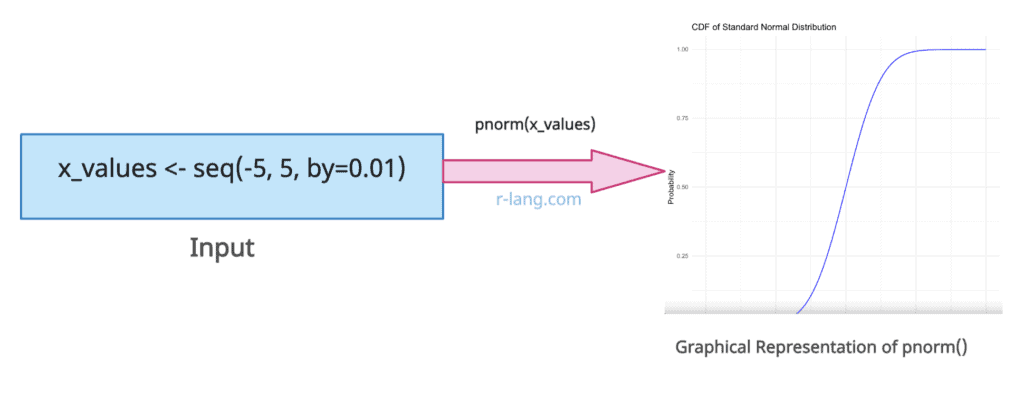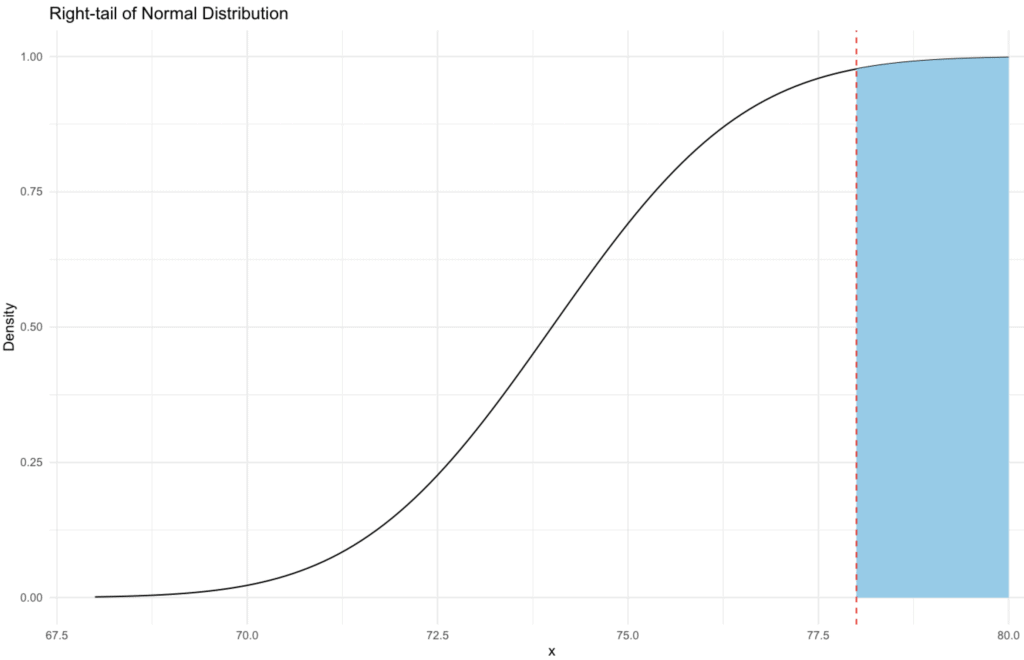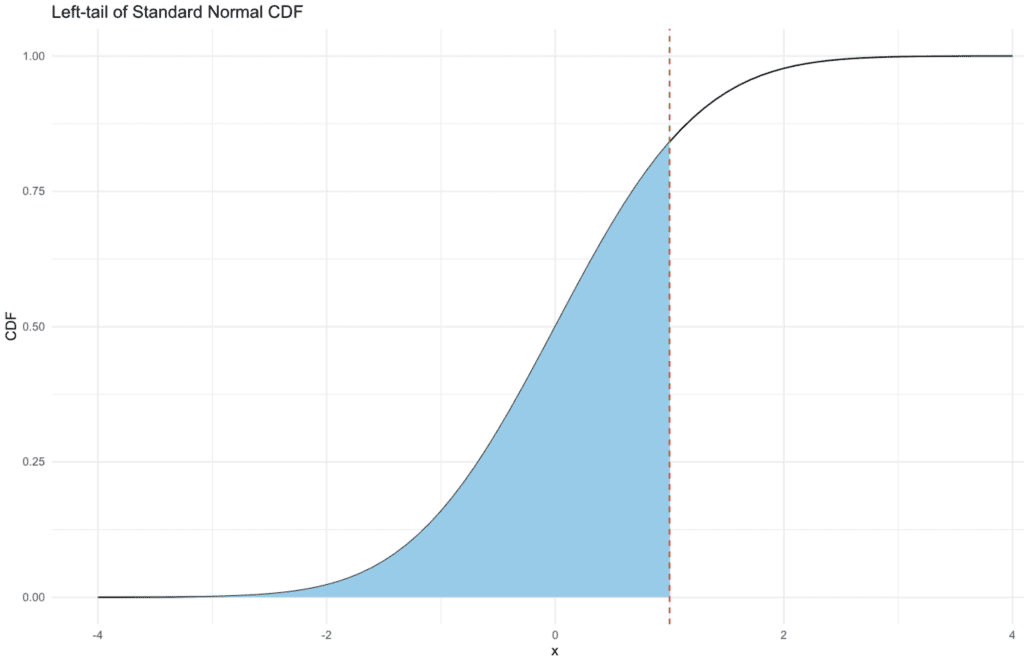# How to Use pnorm() Function in R [With Graphical Representation]

The pnorm() function in R returns the Cumulative Density Function (CDF) value of the normal distribution given a specific random variable q, the population mean μ, and the population standard deviation σ.

##Syntax

``pnorm(q, mean, sd, lower.tail = TRUE, log.p = FALSE)``

## Parameters

1. q: It is a vector of quantiles.
2. mean: It is a vector of means.
3. sd: It is a vector of standard deviations.
4. lower.tail: It is logical; if TRUE (default), probabilities are otherwise.
5. log, log.p: It is a logical argument.

## Return value

It returns the Cumulative Density Function (CDF) value of the normal distribution. If mean or sd are not specified, they assume the default values of 0 and 1, respectively.

## Example 1: How to Use the pnorm() function

Find the percentage of males taller than 78 inches in a population with mean = 74 and sd = 2.

``pnorm(78, mean = 74, sd = 2, lower.tail = FALSE)``

Output

`` 0.02275013``

### Graphical representation

To visualize the pnorm() function, we can plot the probability density function (PDF) of the normal distribution and shade the area to the right of x=78, representing the right-tail probability.

We will use the RStudio to plot the chart and ggplot2 library. Install if you have not already!

``````# Load necessary libraries
library(ggplot2)

# Define parameters
mean_val <- 74
sd_val <- 2

# Create a sequence of x values
x <- seq(68, 80, by = 0.1)

# Calculate the PDF for the x values
y <- pnorm(x, mean = mean_val, sd = sd_val)

# Plot the PDF
p <- ggplot(data.frame(x = x, y = y), aes(x = x, y = y)) +
geom_line() +
geom_area(
data = data.frame(x = x[x >= 78], y = y[x >= 78]),
aes(x = x, y = y), fill = "skyblue"
) +
geom_vline(aes(xintercept = 78), linetype = "dashed", color = "red") +
labs(
title = "Right-tail of Normal Distribution",
x = "x",
y = "Density"
) +
theme_minimal()

# Display the plot
print(p)
``````## Example 2: Using pnorm() with different parameters

``pnorm(33, mean=40, sd = 8)``

Output

`` 0.190787``

### Graphical representation

``````# Load necessary libraries
library(ggplot2)

# Define parameters
mean_val <- 40
sd_val <- 8

# Create a sequence of x values
x <- seq(20, 60, by = 0.1)

# Calculate the CDF for the x values
y <- pnorm(x, mean = mean_val, sd = sd_val)

# Plot the CDF
p <- ggplot(data.frame(x = x, y = y), aes(x = x, y = y)) +
geom_line() +
geom_vline(aes(xintercept = 33), linetype = "dashed", color = "red") +
geom_point(aes(x = 33, y = pnorm(33, mean = mean_val, sd = sd_val)), color = "blue") +
labs(title = "CDF of Normal Distribution",
x = "x",
y = "pnorm(x, mean = 40, sd = 8)") +
theme_minimal()

# Display the plot
print(p)``````## Example 3: Passing the lower.tail = TRUE

``````# Load necessary libraries
library(ggplot2)

# Define parameters
mean_val <- 0
sd_val <- 1

# Create a sequence of x values
x <- seq(-4, 4, by = 0.1)

# Calculate the CDF for the x values
y <- pnorm(x, mean = mean_val, sd = sd_val, lower.tail = TRUE)

# Plot the CDF
p <- ggplot(data.frame(x = x, y = y), aes(x = x, y = y)) +
geom_line() +
geom_area(data = data.frame(x = x[x <= 1], y = y[x <= 1]),
aes(x = x, y = y), fill = "skyblue") +
geom_vline(aes(xintercept = 1), linetype = "dashed", color = "red") +
labs(title = "Left-tail of Standard Normal CDF",
x = "x",
y = "CDF") +
theme_minimal()

# Display the plot
print(p)``````## Example 4: Calculating pnorm() with a mean of 0

``````# Import the stats package
library(stats)

# Calculate the cumulative probability of a normal distribution with a mean of 0,
# a standard deviation of 1, and a quantile of 0.5
prob <- pnorm(q = 0.5, mean = 0, sd = 1)

# Print the cumulative probability
print(prob)``````

Output

`` 0.6914625``

## Example 5: Visualization

``````# Load necessary libraries
library(ggplot2)

# Define the range and compute the CDF values
x_values <- seq(-5, 5, by=0.01)
y_values <- pnorm(x_values)
print(y_values)

# Create the plot
plot <- ggplot(data.frame(x=x_values, y=y_values), aes(x=x, y=y)) +
geom_line(color="blue") +
ggtitle("CDF of Standard Normal Distribution") +
xlab("x") +
ylab("Probability") +
theme_minimal()

# Display the plot
plot
``````

OutputThat’s it.